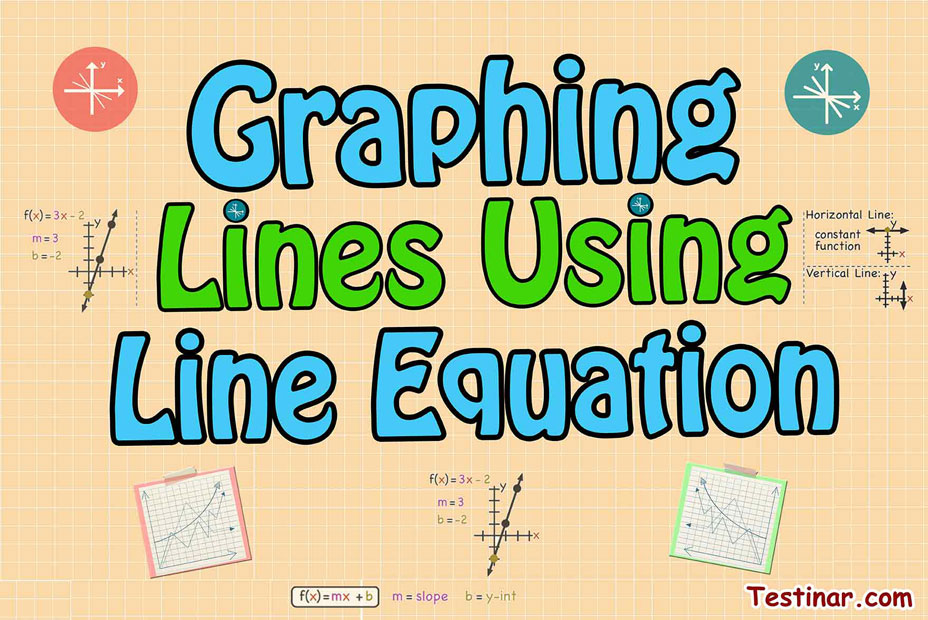## How To Graph Lines Using Line Equation

Graphing lines from equations is a fundamental skill in algebra. It helps us visualize linear equations and solve problems related to them. Here's how you can graph a line from its equation:

## Understanding the Line Equation

The most common form of a line equation is in the slope-intercept form, which is $$y = mx + b$$, where:

• $$m$$ is the slope of the line
• $$b$$ is the y-intercept of the line, which is the point where the line crosses the y-axis.

## Plotting the y-intercept

First, we start by plotting the y-intercept $$(b)$$ on the graph. This point is always on the y-axis and is given by the coordinates $$(0, b)$$.

## Using the Slope to Plot the Line

Next, we use the slope $$m$$ to determine the direction of the line. The slope is the rise (vertical change) over run (horizontal change). A positive slope means the line goes upward to the right, and a negative slope means it goes downward to the right. Starting from the y-intercept, if the slope is $$2/1$$, for example, we can move $$2$$ units up (rise) and $$1$$ unit to the right (run) to find another point on the line.

## Drawing the Line

After plotting the y-intercept and using the slope to find another point, we can draw a straight line through these points. This line represents the solution to the line equation.

## Example

Consider the equation $$y = 2x + 3$$. Here, the slope $$m$$ is $$2$$ and the y-intercept (b) is $$3$$. We can plot the point $$(0, 3)$$ for the y-intercept. Then, we use the slope $$2/1$$ to find that we move $$2$$ units up and $$1$$ unit to the right to plot another point $$(1, 5)$$. Drawing a line through these points gives us the graph of the line $$y = 2x + 3$$.

## Graphing Lines Using Line Equation Quiz

### The Most Comprehensive ATI TEAS 6 Math Preparation Bundle

$76.99$36.99

### AFOQT Math for Beginners

$24.99$14.99

### AFOQT Math Practice Workbook

$25.99$14.99

### FTCE Math Study Guide 2020-2021

$20.99$14.99